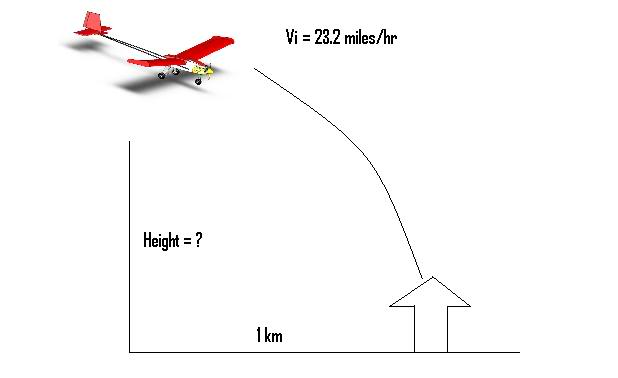# How to find height in Projectile Motion?

Hello guys!

I need your help regarding Projectile Motion because I kinda novice here. I don't know where to start.

These are the following given:
Vi = 23.2 miles/hour
h = ?
x = 1 km

Here's the situation it looks like, check this image that I created for you to understand.Hope someone can help me with this.

Thanks and God bless. :)

## The Attempt at a Solution

For a start you'd need to have an angle of projection, and convert all figures into the same system. The diagram isn't over clear to me, as it seems your plane is pointing away from the projection (but it is early here)

Unit are not the same here!!!
You need to find time.
Use these formulae you can get the answer. Don't forget to change same unit here. SI and British Unit!!!
t=x/Vi
h=0.5gt^2

remember that the velocity in x direction is constant then you can use the x component velocity to find time by D=VT then solve for height in y direction

Like seto6 said, the acceleration in X is 0. You can find the time that you will need to resolv the Y equation.

Actually, All of us(seto6, proculation and inky) method are the same.
v[0x]=v[x]=v
v[0y]=0

t=x/v=1/(23.2*1.6)=0.0269 hr=96.84 s
h=y=v[0y]t+0.5gt=0+0.5(10)(96.84^2)=46890m=46.89 km

Last edited: